Equivalent summation methods

Methods that sum the same sequences (possibly to different limits); in other words, equivalent summation methods are summation methods having the same summability field. Sometimes methods that have the same summability field and that are compatible summation methods (cf. Compatibility of summation methods) are called equivalent. Examples of equivalent and compatible methods are the Cesàro summation methods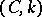and the Riesz summation methods (cf. Riesz summation method)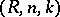(for the same), the Cesàro summation methodsand the Hölder summation methods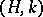(for the same integer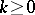). There are equivalent summation methods that are not compatible.
Sometimes one considers not the complete summability fields, but subsets of them belonging to some set. If these subsets coincide for two summation methods, then it is said that the methods are equivalent on. Summation methods for real sequences are called completely equivalent if the equality of their summability fields remains valid upon the inclusion of sequences summable toand. Equivalence of summation methods for special forms of summability (absolute, strong, etc.) is defined similarly.
Matrix summation method, defined by transformations of sequences to sequences using matrices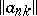and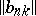, are called absolutely equivalent on a setof sequences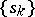if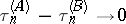,, for any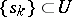, whereand the series in the expressions for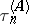and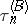converge for all.